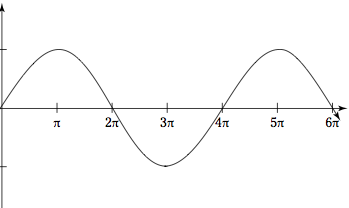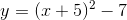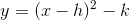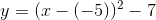# Advanced Geometry : How to find transformation for an analytic geometry equation

## Example Questions

1 3 Next →

### Example Question #9 : Transformation

Let f(x) = x3 – 2x2 + x +4. If g(x) is obtained by reflecting f(x) across the y-axis, then which of the following is equal to g(x)?

x3 + 2x2 + x + 4

–x3 – 2x2 – x + 4

–x3 – 2x2 – x – 4

–x3 + 2x2 – x + 4

x3 – 2x2 – x + 4

–x3 – 2x2 – x + 4

Explanation:

In order to reflect a function across the y-axis, all of the x-coordinates of every point on that function must be multiplied by negative one. However, the y-values of each point on the function will not change. Thus, we can represent the reflection of f(x) across the y-axis as f(-x). The figure below shows a generic function (not f(x) given in the problem) that has been reflected across the y-axis, in order to offer a better visual understanding.Therefore, g(x) = f(–x).

f(x) = x3 – 2x2 + x – 4

g(x) = f(–x) = (–x)3 – 2(–x)2 + (–x) + 4

g(x) = (–1)3x3 –2(–1)2x2 – x + 4

g(x) = –x3 –2x2 –x + 4.

The answer is –x3 –2x2 –x + 4.

### Example Question #5 : How To Find Transformation For An Analytic Geometry Equation

What is the period of the function?2π

π

3π

1

4π

4π

Explanation:

The period is the time it takes for the graph to complete one cycle.

In this particular case we have a sine curve that starts at 0 and completes one cycle when it reaches.

Therefore, the period is### Example Question #231 : Coordinate Geometry

Explain how the below function translates:5 units left, 7 units down

5 units up, 7 units left

5 units down, 7 units right

5 units right, 7 units down

5 units left, 7 units down

Explanation:

When estimating the translations for a quadratic function we must remember what vertex form for a parabola looks like:In order to end up with:We must have the below to end up with a positive 5:This is what gives us the translation left 5 spaces and down 7 spaces.

### Example Question #232 : Coordinate Geometry

Assume we have a triangle,, with the following vertices:, andIfwere reflected across the line, what would be the coordinates of the new vertices?Explanation:

When we reflect a point across the line,, we swap the x- and y-coordinates; therefore, in each point, we will switch the x and y-coordinates:becomesbecomes, andbecomesThe correct answer is the following:The other answer choices are incorrect because we only use the negatives of the coordinate points if we are flipping across either the x- or y-axis.

1 3 Next →